## Main

Control of single photonic qubits using linear optics has been an appealing approach to implementing quantum computing6. Experiments in recent years have demonstrated the photons’ extremely long decoherence time7, fast clock speed8, a series of controlled quantum logic gates9,10 and algorithms8,11,12 and the generation of multiqubit entangled states5. A significant challenge, however, lies in making experimentally accessible sources of photonic multi-qubit states. This is because, on the one hand, the probabilistic nature of spontaneous parametric down-conversion13 represents a bottleneck with regard to the attainable brightness and fidelity of multiphoton states based on it: manipulating seven photons or more seems an insurmountable challenge with present technology. On the other hand, triggered single-photon sources from independent quantum dots or other emitters still suffer from spectral and temporal distinguishability, which prevents up-scaling.

There is, however, a way to experimentally control more effectively qubits, by exploiting hyper-entanglement14—the simultaneous entanglement in the multiple degrees of freedom that naturally exist for various physical systems. For instance, one can encode quantum information not only in the polarization of a single photon, but also in its spatial modes15, arrival time15 or orbital angular momentum16. Recently, hyper-entangled photonic states15 have been experimentally realized, and shown to offer significant advantages in quantum super-dense coding17,18, enhanced violation of local realism19, efficient construction of cluster states20,21 and multiqubit logic gates10.

Although the largest hyper-entangled state15 realized so far has expanded the Hilbert space up to 144 dimensions, it is a product state of two-party entangled states and does not involve multipartite entanglement. Other schemes20,21 for creating hyper-entanglement have been limited by the technical problem of photonic subwavelength phase stability and seem infeasible to generate larger states than the two-photon four-qubit ones. Here, we will describe a method that overcomes these limitations, and the experimental generation of hyper-entangled six-, eight- and ten-qubit photonic Schrödinger cat states.

The Schrödinger cat states, also technically known as Greenberger–Horne–Zeilinger states22, involve an equal superposition of two maximally different quantum states. They are of particular interest in quantum mechanics and find wide applications in quantum information processing. Our experiment aims to create the cat state in the form: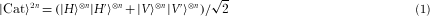where H and V denote horizontal and vertical polarization and H′ and V ′ label two orthogonal spatial modes (or momentums) of the photons. The state (1) shows maximal entanglement between all photons’ polarization and spatial qubits.

Our first experimental step is to generate polarization-entangled n-qubit cat states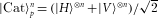. Two pairs of entangled photons are produced by spontaneous parametric down-conversion13 in the state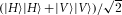in paths 2–3 and 4–5, and a pseudo-single photon source23 is prepared in the polarization state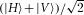in path 1 (see Fig. 1a and the Methods section). Photons 3 and 4 are superposed on a polarizing beam splitter (PBS1), and then are further combined with photon 1 on PBS2. Fine adjustments of the delay between the different paths are made to ensure that the photons arrive at the PBSs simultaneously. Furthermore, the photons are spectrally filtered and detected by single-mode fibre-coupled single-photon detectors for good spatial and temporal overlap. As the PBSs transmit H and reflect V polarization, it can be concluded that a coincidence detection of the five output photons implies that all of the photons are either H or V polarized—the two cases are quantum mechanically indistinguishable—therefore projecting them in the cat state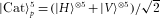. It is easy to check that, in a similar way, if we combine only photons 1 and 4 (3 and 4) on PBS2 (PBS1), entangled cat states between the three photons 1–4–5 (the four photons 2–3–4–5) can be created by post-selection.Figure 1: Experimental set-up for the generation of hyper-entangled six-, eight- and ten-qubit Schrödinger cat states.

Next, we grow the polarization-encoded n-qubit states into double-sized 2n-qubit cat states by planting spatial modes on them. This again exploits the PBSs. Consider a polarized single-photon qubit in the state α|H〉+β|V 〉 that passes through a PBS (see Fig. 1b). The PBS separates the photon into two possible spatial modes H′ and V ′, according to their polarization H and V respectively; indeed, this forms the basis of the PBS as an instrument for measuring polarization. The state of this single photon can now be written as α|H〉|H′〉+β|V 〉|V ′〉, an entangled state between its polarization and spatial degree of freedom. It is straightforward to extend this method to the n-photon state |Cat〉pn; thereby, the hyper-entangled 2n-qubit cat state (1) can be created. Besides the cat states, we note that this method can also be flexibly modified for the generation of other graph states24, which are central resources in measurement-based quantum computing (see Supplementary Fig. S1).

With multiple degrees of freedom carrying the quantum information in a single photon, measurements of the composite quantum states now become a bit trickier, as it is necessary to read out one degree of freedom without disturbing another one. Figure 1b illustrates the apparatus for simultaneously yet independently measuring both the polarization and spatial qubits on the basis of |0〉/|1〉 and (|0〉±eiθ|1〉) (here we denote |H〉=|H′〉 as logic |0〉 and |V 〉=|V ′〉 as |1〉). Specifically, the measurement of the spatial qubit uses an optical interferometer combing the two paths onto a non-polarizing beam splitter (NBS) with an adjustable delay between these two paths that controls the relative phase θ. After this interferometer, the polarization information is then read out by using a combination of a quarter-wave plate, a half-wave plate and a PBS in front of the single-photon detectors, projecting the polarization states into (|H〉±eiθ|V 〉). Experimentally, however, it is difficult to directly implement the interferometer in Fig. 1b, because it is sensitive to path length instability of the order of the photon’s wavelength. To overcome this problem, we construct intrinsically stable Sagnac-like interferometers with beam-splitter cubes that are half PBS-coated and half NBS-coated (see Fig. 1c). The long-term stabilities and the high visibilities of the five interferometers constructed in our experiment are shown in Supplementary Fig. S2.

As a step-by-step approach, we begin with the creation of the hyper-entangled six-qubit cat state |Cat〉6 and eight-qubit cat state |Cat〉8. To analyse the experimentally produced states, we first look at the measurement results in the |0〉/|1〉 basis, as shown in Fig. 2a,b for |Cat〉6 and |Cat〉8, respectively. For ideal cat states, the desired combinations in this basis should in principle be only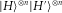and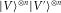. This is confirmed by the experimental data in Fig. 2a,b, showing that these two terms dominate the overall coincidence events, with a signal-to-noise ratio (defined as the ratio of the average of the desired components to that of the other non-desired ones) of 85:1 to 1,100:1 for the states |Cat〉6 and |Cat〉8, respectively. We note that the undesired noise, noticeably located in the diagonal line of Fig. 2a,b, mainly arises from the double-pair emission of entangled photons.Figure 2: Experimental results for determination of the fidelities of the six- and eight-qubit cat states, showing phase super-resolution.

Although the above data determine the population in the |0〉/|1〉 basis of the cat states, they are not sufficient to reveal their coherence properties. We took therefore additional measurements in the basis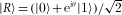and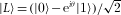. In this new basis, the cat state |Cat〉n can be written in the form of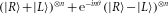; thus, the probabilities of creating the components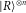,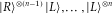and hence the experimentally observed coincidence events should vary as (1±cosn θ). From these measurements, one can determine the expectation values of the spin observable: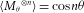, where Mθ=cosθ σx+sinθ σy, which oscillates n times sinusoidally over a single cycle of 2π. Indeed, this can arise only from coherent superposition between the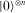and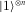component of the cat state and serves as a characteristic signature of n-qubit coherence3.

Figure 2c,d shows the experimentally obtained expectation values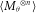as a function of θ (0≤θ≤2π) and the fitted sinusoidal fringes. The fringes clearly show the n θ oscillation, with a visibility of 0.527±0.002 and 0.67±0.01 for the six- and eight-qubit cat states, respectively, confirming the coherence between all effective n qubits encoded with either polarization or spatial information. We note that the reduction of the visibilities is caused by, besides the above-mentioned double-pair photon emission, also the imperfections of photon overlapping at the PBSs and NBSs.

From the data shown in Fig. 2, we can further determine the fidelities of the cat states and detect the presence of genuine multipartite entanglement25. The fidelity—a measure of the extent to which the desired state is created—is the overlap of the experimentally produced state with the ideal one: F(|ψ〉)=〈ψ|ρexp|ψ〉. For the cat state, |ψ〉〈ψ| can be decomposed as5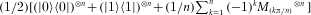, corresponding to measurements in the basis of |0〉/|1〉 and (|0〉±ei(kπ/n)|1〉). Figure 2a–d shows the experimental results, from which the fidelities of the six- and eight-qubit cat states can be determined: F(|Cat〉6)=0.6308±0.0015, F(|Cat〉8)=0.776±0.006. The notion of genuine multipartite entanglement characterizes whether generation of the state requires interaction of all parties, distinguishing the experimentally produced state from any incompletely entangled state. For cat states, it is sufficient for the presence of genuine multipartite entanglement that their fidelities exceed the threshold of 0.5 (ref. 25). Thus, with high statistical significance, genuine n-qubit entanglement of the cat states created in our experiment is confirmed. We note that the fidelity of the six-qubit cat state is considerably lower than that of the eight-qubit state, which is due to the fact that the generation of the former involves a faint coherent laser light, which introduces more noise than the configuration of the latter. It is worth mentioning here an advantage that the hyper-entanglement brings—our new six-qubit cat state not only has a higher fidelity than the previous six-photon cat state5, but also its count rate reaches 200 s−1, some 4 orders of magnitude brighter than the six-photon coincidence.

The fringes in Fig. 2c,d show n θ phase dependencies, which are n times more sensitive to phase changes than that of a single qubit, highlighting the potential use of the cat states for super-resolving phase measurements2. Suppose we need to estimate the phase ϕ of a process |0〉→|0〉 and |1〉→eiϕ|1〉. A simple initial probe state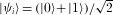would evolve into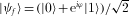and the phase can be estimated from the overlap |〈ψi|ψf〉|2=[1+cos(ϕ)]/2. Provided with N probe states, the uncertainty of the phase estimation is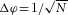—the standard quantum limit. Applying the same dynamics on N qubits in a cat state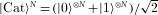, it will evolve to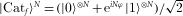. Then, measuring the overlap or the mean value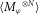leads to dependence on cos(N ϕ), from which the phase can be determined with an error of Δϕ=1/N (refs 2, 3, 26). The 1/N scaling of the precision is called the Heisenberg limit, as it coincides with the limit imposed by the uncertainty principle. As shown in Fig. 2c,d, we have observed the expected n-times phase super-resolution for the six- and eight-qubit cat states, with a visibility of 0.527±0.002 and 0.67±0.01, respectively. These values are larger than the threshold of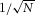for phase super-resolution, and taking into account the cat-state preparation efficiency of 1/2 caused by the PBS filtering (the efficiency can be improved to unity in principle6), we find that the eight-qubit cat state can achieve a phase sensitivity 1.13±0.02 times greater than the standard quantum limit (see Supplementary Information). This represents entanglement-enhanced quantum metrology with the largest number of qubits so far. However, as with all photonic experiments27, the single-photon detection efficiency needs to be improved significantly for a practical implementation of Heisenberg-limited measurements.

Now we proceed with describing the generation and analysis of the ten-qubit cat state |Cat〉10, which uses the full set-up shown in Fig. 1. As a result of the probabilistic nature of spontaneous parametric down-conversion, the coincidence count rate of the ten-qubit state is as low as 0.021 Hz, 1/160 (1/11,000) of that of the eight-qubit (six-qubit) state. Measurement results along the |0〉/|1〉 basis are shown in Fig. 3a with all 210=1,024 possible combinations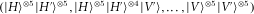plotted, giving a signal to noise ratio (defined as above) of 940:1. We further take measurements in the (|0〉±eiθ|1〉) basis, where θ is chosen as: θ=kπ/10, k=0,1,…,9. The measured expectation values of the observable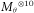are listed in Fig. 3b with an average absolute value of 0.475—this can also be seen equivalently as the fringe visibility shown in Fig. 2c,d. We can thus calculate the state fidelity: F(|Cat〉10)=0.561±0.019, which is above the threshold of 0.5 by more than three standard deviations, thus establishing the presence of genuine ten-qubit entanglement after post-selection. These data are further analysed using an optimized entanglement witness method (see Supplementary Information), which, with even higher significance, confirms that the entanglement truly involves all ten qubits.

The photon pairs and single photons for the later observation of the cat state are generated as follows: as shown in Fig. 1a, a femtosecond infrared laser is attenuated to be a weak coherent photon source that has a very small probability (p0.03) of containing a single photon for each pulse, and prepared in the superposition state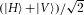in path 1. Meanwhile, a pulsed ultraviolet (ultraviolet) laser, which is up-converted from the intense infrared laser, passes through two β-barium borate crystals, generating two pairs of entangled photons in the state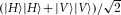in paths 2–3 and 4–5. The photon pairs have an average two-photon coincidence count rate of 2.4×104 s−1 and a visibility of 0.92 in the H/V basis and 0.90 in the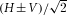basis.